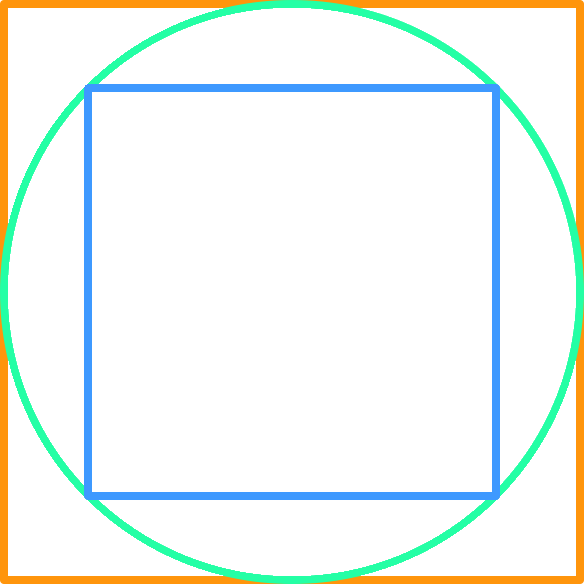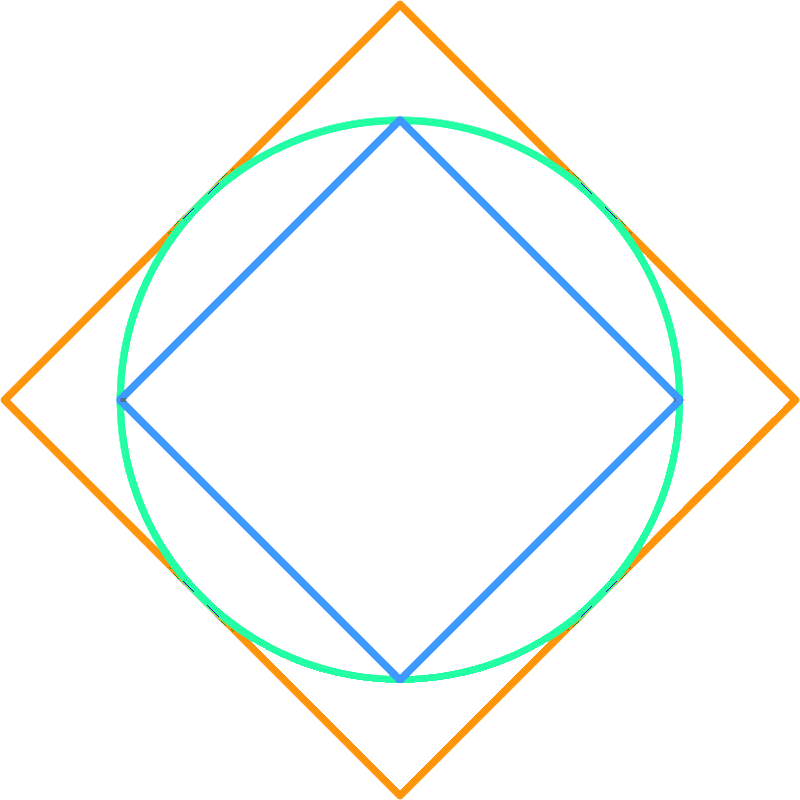cd ~

# How Big of a Square Fits in a Circle?

Turns out you can fit a square peg into a round hole. I learned this while building an app.My app required a circular button (green circle in the diagram) with a square image inside (blue square). The circular button was itself a square image (orange square), just with the parts outside the circle left transparent.

How big could the image be, and still fit inside the circular button? In terms of the diagram above, how big can the blue square be, while fitting inside the green circle?When you rotate the picture, you can see that the diameter of the circle is the same as the diagonal length across the blue square. (This is also the same as the length of each side of the orange square.).

Call the blue square’s diagonal length d, and its side length s. Then, by the Pythagorean Theorem,

$d^2 = s^2 + s^2$
$d^2 = 2s^2$

Take the square root of both sides.

$d = \sqrt{2s^2}$
$\frac{d}{\sqrt{2}} = s$

When a square is inscribed within a circle, the side length of the square is equal to the diameter of the circle, divided by the square root of 2.